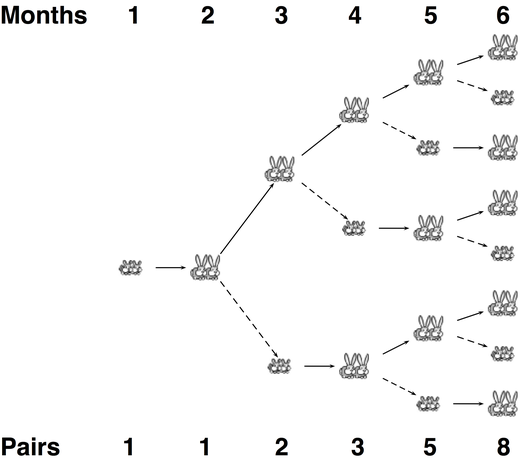# Glossary

## Fibonacci sequence

The Fibonacci sequence is a sequence of numbers defined by the recurrence relation $F_n = F_{n-1} + F_{n-2}$, where we set the starting values $F_1 = F_2 = 1$.

The Fibonacci sequence appears throughout the natural world, and it dates back over two millennia to its first use by Indian mathematicians; yet its name derives from Leonardo of Pisa, who popularized the sequence in the West by introducing it as an exercise involving a population of rabbits in 1202. His assumption about the population were as follows:

1. The population begins in the first month with a pair of newborn rabbits.
2. Rabbits reach reproductive age in one month.
3. In any month, every rabbit of reproductive age mates with another rabbit of reproductive age.
4. One month after two rabbits have mated, the female rabbit gives birth to a male and female rabbit.
5. Rabbits never stop reproducing or die.

See the figure below for a branching diagram illustrating the number of rabbit pairs for each of the first five months. The dynamics of the rabbit population explains the recurrence relation $F_n = F_{n-1} + F_{n-2}$, as in the $n$-th month, the total number of rabbits will equal the number of rabbits alive the previous month ($F_{n-1}$) plus the number of newborn rabbits, which is equal to the number of adult rabbits, or the number of rabbits alive two months previously ($F_{n-2}$).

The first 12 terms of the Fibonacci sequence are 1, 1, 2, 3, 5, 8, 13, 21, 34, 55, 89, 144. The 12th term (144) gives the number of rabbits after one year, which answers Fibonacci's original question to his readers.